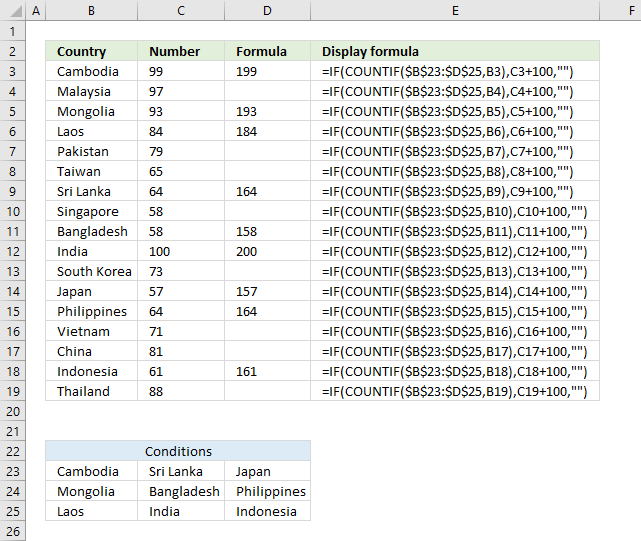Author: Oscar Cronquist Article last updated on March 25, 2018The COUNTIF function allows you to construct a small IF formula that carries out plenty of logical expressions.

Combining the IF and COUNTIF functions also let you have more than 254 logical expressions and the effort to type the formula is minimal.

=IF(COUNTIF(\$B\$23:\$D\$25,B3),C3+100,"")

The example shown in above picture checks if the country in cell B3 is equal to one of the countries in cell range B23:D25.

In other words, the COUNTIF function counts how many times a specific value is found in a cell range.

If the value exists at least once in the cell range the IF function adds 100 to the value in C3. If FALSE the formula returns a blank.

COUNTIF(\$B\$23:\$D\$25,B3)

becomes

COUNTIF({"Cambodia", "Sri Lanka", "Japan"; "Mongolia", "Bangladesh", "Philippines"; "Laos", "India", "Indonesia"}, "Cambodia")

and returns 1. The criteria value is found once in the array (bolded).

IF(COUNTIF(\$B\$23:\$D\$25, B3), C3+100, "")

The IF function interprets 1 as TRUE and carrys out the value_if_true argument, C3+100.

IF(1,99+100, "")

C3 is 99 and 99 + 100 equals 199, the IF function returns 199 in cell D3.